Home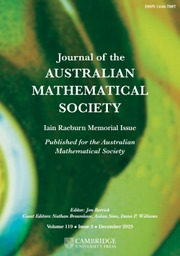Journal of the Australian Mathematical Society

# b-GENERALIZED DERIVATIONS OF SEMIPRIME RINGS HAVING NILPOTENT VALUES

Published online by Cambridge University Press:  31 March 2014

Corresponding

## Abstract

Let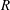$\def \xmlpi #1{}\def \mathsfbi #1{\boldsymbol {\mathsf {#1}}}\let \le =\leqslant \let \leq =\leqslant \let \ge =\geqslant \let \geq =\geqslant \def \Pr {\mathit {Pr}}\def \Fr {\mathit {Fr}}\def \Rey {\mathit {Re}}R$ be a semiprime ring with extended centroid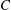$C$ and with maximal right ring of quotients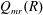$Q_{mr}(R)$ . Let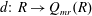$d{:}\ R\to Q_{mr}(R)$ be an additive map and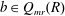$b\in Q_{mr}(R)$ . An additive map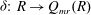$\delta {:}\ R\to Q_{mr}(R)$ is called a (left)$b$ -generalized derivation with associated map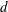$d$ if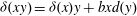$\delta (xy)=\delta (x)y+bxd(y)$ for all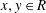$x, y\in R$ . This gives a unified viewpoint of derivations, generalized derivations and generalized$\sigma$ -derivations with an X-inner automorphism$\sigma$ . We give a complete characterization of$b$ -generalized derivations of$R$ having nilpotent values of bounded index. This extends several known results in the literature.

Type
Research Article
Information

## References

Beidar, K. I., Martindale III, W. S. and Mikhalev, A. V., Rings with Generalized Identities, Monographs and Textbooks in Pure and Applied Mathematics, 196 (Marcel Dekker, Inc, New York, 1996).Google Scholar
Brešar, M., ‘On the distance of the composition of two derivations to the generalized derivations’, Glasg. Math. J. 33(1) (1991), 8993.CrossRefGoogle Scholar
Chang, J. C., ‘Generalized skew derivations with nilpotent values on Lie ideals’, Monatsh. Math. 161(2) (2010), 155160.CrossRefGoogle Scholar
Chang, J. C., ‘Generalized skew derivations with power central values on Lie ideals’, Comm. Algebra 39(6) (2011), 22412248.CrossRefGoogle Scholar
Chang, J. C. and Lin, J. S., ‘(α, β)-derivations with nilpotent values’, Chinese J. Math. 22(4) (1994), 349355.Google Scholar
Chuang, C.-L., ‘GPIs having coefficients in Utumi quotient rings’, Proc. Amer. Math. Soc. 103 (1988), 723728.CrossRefGoogle Scholar
Chuang, C.-L. and Lee, T.-K., ‘Derivations modulo elementary operators’, J. Algebra 338 (2011), 5670.CrossRefGoogle Scholar
Erickson, T. S., Martindale III, W. S. and Osborn, J. M., ‘Prime nonassociative algebras’, Pacific J. Math. 60(1) (1975), 4963.CrossRefGoogle Scholar
Faith, C. and Utumi, Y., ‘On a new proof of Litoff’s theorem’, Acta Math. Acad. Sci. Hung. 14 (1967), 369371.CrossRefGoogle Scholar
Giambruno, A. and Herstein, I. N., ‘Derivations with nilpotent values’, Rend. Circ. Mat. Palermo (2) 30(2) (1981), 199206.CrossRefGoogle Scholar
Herstein, I. N., Topics in Ring Theory (The University of Chicago Press, Chicago, Ill.-London, 1969).Google Scholar
Herstein, I. N., ‘Center-like elements in prime rings’, J. Algebra 60 (1979), 567574.CrossRefGoogle Scholar
Herstein, I. N., ‘Derivations of prime rings having power central values’, in: Algebraists’ Homage: Papers in Ring Theory and Related Topics (New Haven, Conn., 1981), Contemporary Mathematics, 13 (American Mathematical Society, Providence, RI, 1982), 163171.CrossRefGoogle Scholar
Hvala, B., ‘Generalized derivations in rings’, Comm. Algebra 26(4) (1998), 11471166.CrossRefGoogle Scholar
Kharchenko, V. K., ‘Differential identities of semiprime rings’, Algebra Logika 18 (1979), 86119; Algebra Logic 18 (1979), 58–80 (English translation).CrossRefGoogle Scholar
Lanski, C., ‘Derivations with nilpotent values on left ideals’, Comm. Algebra 22(4) (1994), 13051320.CrossRefGoogle Scholar
Lee, T.-K., ‘Semiprime rings with differential identities’, Bull. Inst. Math. Acad. Sin. 20 (1992), 2738.Google Scholar
Lee, T.-K., ‘Generalized derivations of left faithful rings’, Comm. Algebra 27(8) (1999), 40574073.CrossRefGoogle Scholar
Lee, T.-K., ‘Generalized skew derivations characterized by acting on zero products’, Pacific J. Math. 216(2) (2004), 293301.CrossRefGoogle Scholar
Lee, T.-K. and Liu, K.-S., ‘The Skolem-Noether theorem for semiprime rings satisfying a strict identity’, Comm. Algebra 35(6) (2007), 19491955.CrossRefGoogle Scholar
Lee, T.-K. and Liu, K.-S., ‘Generalized skew derivations with algebraic values of bounded degree’, Houston J. Math. 39(3) (2013), 733740.Google Scholar
Lee, T.-K. and Zhou, Y., ‘An identity with generalized derivations’, J. Algebra Appl. 8(3) (2009), 307317.CrossRefGoogle Scholar
Martindale III, W. S., ‘Prime rings satisfying a generalized polynomial identity’, J. Algebra 12 (1969), 576584.CrossRefGoogle Scholar
Xu, X., Ma, J. and Niu, F., ‘Annihilators of power central values of generalized derivations’, Adv. Math. (China) 41(1) (2012), 113119.Google Scholar

### Full text views

Full text views reflects PDF downloads, PDFs sent to Google Drive, Dropbox and Kindle and HTML full text views.

Total number of HTML views: 0
Total number of PDF views: 143 *
View data table for this chart

* Views captured on Cambridge Core between September 2016 - 26th January 2021. This data will be updated every 24 hours.

Access
Hostname: page-component-898fc554b-p5tlp Total loading time: 0.289 Render date: 2021-01-26T10:32:25.960Z Query parameters: { "hasAccess": "1", "openAccess": "0", "isLogged": "0", "lang": "en" } Feature Flags: { "shouldUseShareProductTool": true, "shouldUseHypothesis": true, "isUnsiloEnabled": true, "metricsAbstractViews": false, "figures": false, "newCiteModal": false }

# Send article to Kindle

Note you can select to send to either the @free.kindle.com or @kindle.com variations. ‘@free.kindle.com’ emails are free but can only be sent to your device when it is connected to wi-fi. ‘@kindle.com’ emails can be delivered even when you are not connected to wi-fi, but note that service fees apply.

Find out more about the Kindle Personal Document Service.

b-GENERALIZED DERIVATIONS OF SEMIPRIME RINGS HAVING NILPOTENT VALUES
Available formats
×

# Send article to Dropbox

To send this article to your Dropbox account, please select one or more formats and confirm that you agree to abide by our usage policies. If this is the first time you use this feature, you will be asked to authorise Cambridge Core to connect with your <service> account. Find out more about sending content to Dropbox.

b-GENERALIZED DERIVATIONS OF SEMIPRIME RINGS HAVING NILPOTENT VALUES
Available formats
×

# Send article to Google Drive

To send this article to your Google Drive account, please select one or more formats and confirm that you agree to abide by our usage policies. If this is the first time you use this feature, you will be asked to authorise Cambridge Core to connect with your <service> account. Find out more about sending content to Google Drive.

b-GENERALIZED DERIVATIONS OF SEMIPRIME RINGS HAVING NILPOTENT VALUES
Available formats
×
×